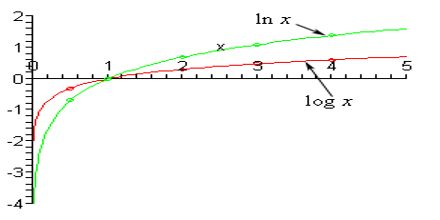Mathematic

# Logarithmic FunctionsBasic objective of this lecture is to present on Logarithmic Functions. Since is a one-to-one function, we know that its inverse will also be a function. When we graph the inverse of the natural logarithmic function, we notice that we obtain the natural exponential function, f (x) = ex. Notice how (1,0) from y = ln x becomes (0,1) for f (x) = ex. Hee also describe on types of logarithms: logarithms to base 10 are called common logarithms; logarithms to base 2 are called binary logarithms and logarithms to base ‘e’ are called natural logarithms.